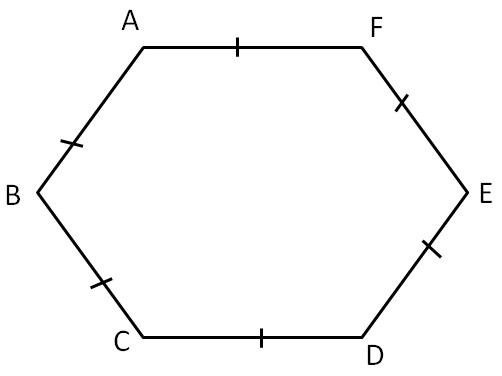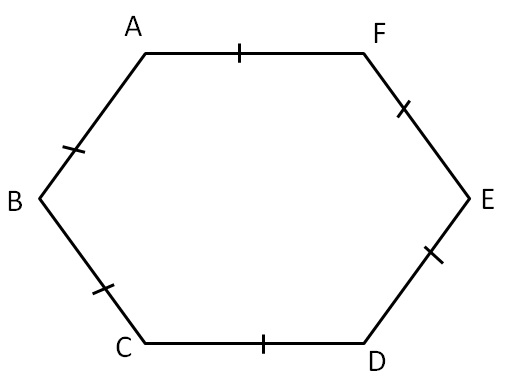Sum of Exterior Angles of PolygonsIn a regular polygon

• All sides are equal
• All angles are equal
•

Let

∠ A = ∠ B = ∠ C = ∠ D = ∠ E = ∠ F = x

We know that

Sum of angles of a regular hexagon = (n – 2)  × 180°

Putting n = 6

= (6 – 2) × 180°

= 4 × 180°

= 720°

Now,

Sum of angles of a regular hexagon= 720°

∠ A + ∠ B + ∠ C + ∠ D + ∠ E + ∠ F = 720°

x + x + x + x + x + x = 720°

6x = 720°

x = (720°)/6

x = 120°

Thus,

Interior Angle of a Regular Hexagon = 120°In general

Interior Angle of a Polygon × Number of sides = Sum of angles

Interior Angle of a Regular Polygon  × n = (n – 2) × 180°

Interior Angle of a Regular Polygon  = ((n - 2))/n   × 180°

Learn in your speed, with individual attention - Teachoo Maths 1-on-1 Class